# Linear equation forms questions and answers

Recent questions in Forms of linear equationsankarskogC 2021-09-14 Answered

### The reduced row echelon form of the augmented matrix of a system of linear equations is given. Tell whether the system has one solution, no solution, or infinitely many solutions. Write the solutions or, if there is no solution, say the system is inconsistent. [101 011 000]generals336 2021-09-14 Answered

### The coefficient matrix for a system of linear differential equations of the form y′=Ay has the given eigenvalues and eigenspace bases. Find the general solution for the system. $$\lambda_1=2\Rightarrow\left\{\begin{bmatrix}4 \\3 \\1 \end{bmatrix}\right\},\lambda_2=-2\Rightarrow\left\{\begin{bmatrix}1 \\2 \\0 \end{bmatrix},\begin{bmatrix}2 \\3 \\1 \end{bmatrix}\right\}$$avissidep 2021-09-14 Answered

### Suppose that the augmented matrix for a system of linear equations has been reduced by row operations to the given reduced row echelon form. Solve the system. Assume that the variables are named x1,x2,…x,… form left to right. $$\begin{bmatrix}1 & -3 & 0 & 0 \\0 & 0 & 1 & 0 \\0 & 0 & 0 & 1 \end{bmatrix}$$Lewis Harvey 2021-09-13 Answered

### Determine whether the statement is true or false. If the last row of the reduced row echelon form of an augmented matrix of a system of linear equations has only zero entries, then the system has infinitely many solutions.Khadija Wells 2021-09-13 Answered

### The reduced row echelon form of the augmented matrix of a system of linear equations is given. Determine whether this system of linear equations is consistent and, if so, find its general solution. Write the solution in vector form. $$\begin{bmatrix}1 & 0 & 0 & -3 & 0 \\0 & 1 & 0 & -4 & 0 \\0 & 0 & 1 & 5 & 0 \end{bmatrix}$$Chardonnay Felix 2021-09-13 Answered

### Reduce the system of linear equations to upper triangular form and solve. ​ 7x−y=4 3x+2y=1Chardonnay Felix 2021-08-16 Answered

### The reduced row echelon form of a system of linear equations is given.Write the system of equations corresponding to the given matrix. Use x, y. or x, y, z. or $$x_1, x_2, x_3, x_4$$ as variables. Determine whether the system is consistent or inconsistent. If it is consistent, give the solution. $$\begin{bmatrix}1 & 0 & 0 & 0 & 1\\ 0 &1 & 0 &0 & 2 \\ 0 & 0 & 1 & 2 & 3 \end{bmatrix}$$hexacordoK 2021-08-14 Answered

### Consider the system (*) whose coefficient matrix A is the matrix D listed in Exercise 46 and whose fundamental matrix was computed just before the preceding exercise.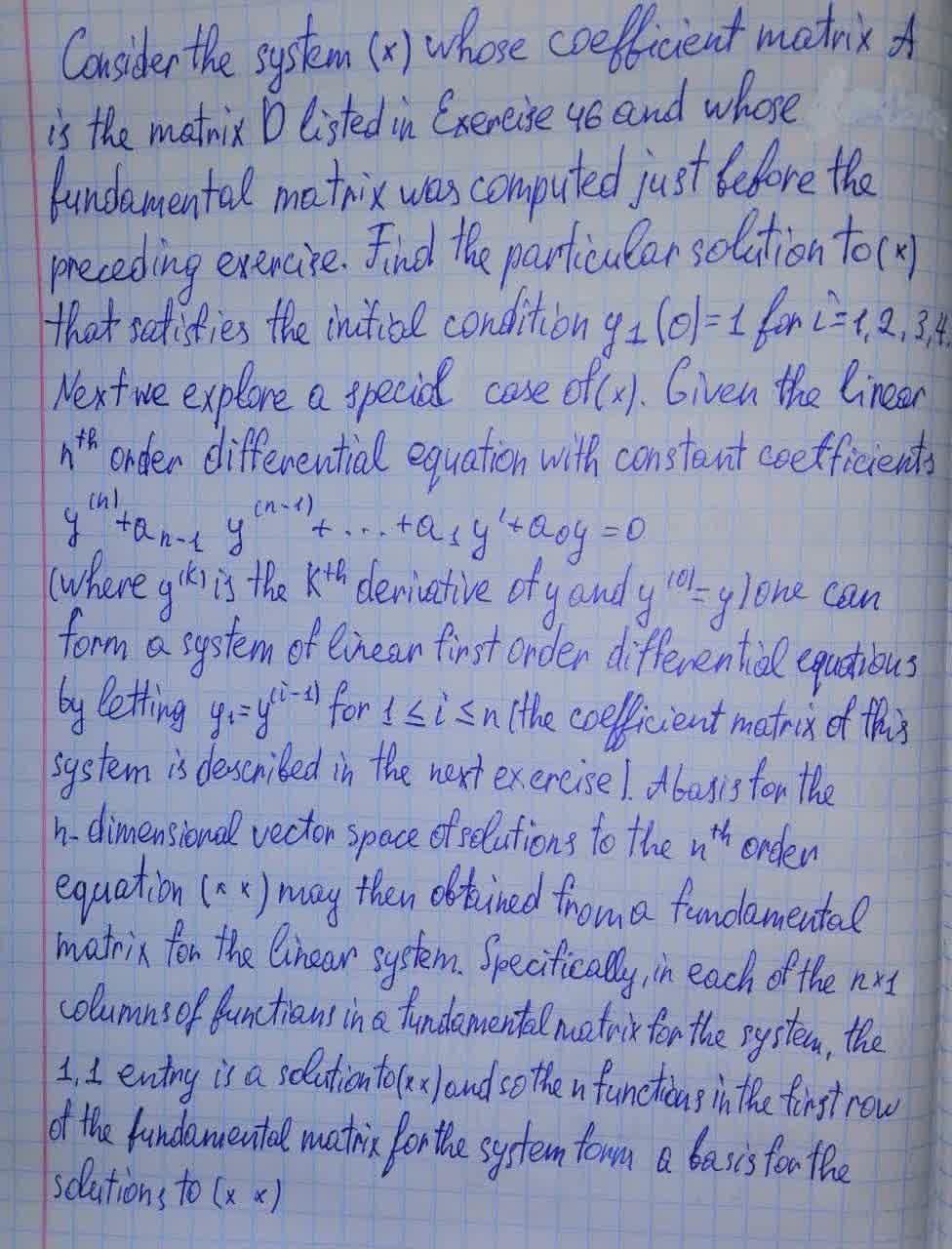CheemnCatelvew 2021-08-13 Answered

### Write the vector form of the general solution of the given system of linear equations. $$\displaystyle-{x}_{{1}}+{2}{x}_{{3}}-{5}{x}_{{4}}+{x}_{{5}}-{x}_{{6}}={0}$$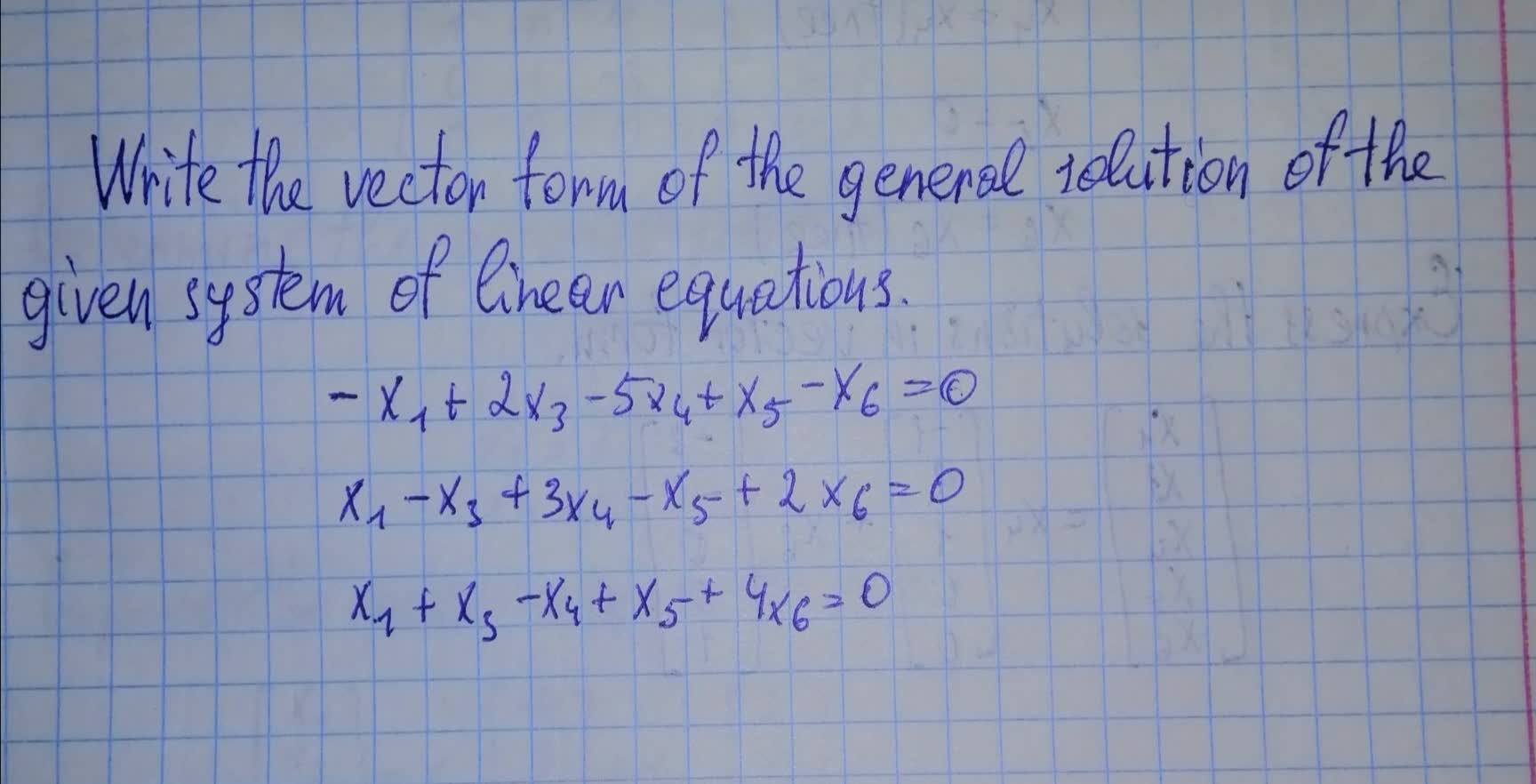preprekomW 2021-08-12 Answered

### The row echelon form of a system of linear equations is given. a) Write the system of equations corresponding to the given matrix. Use x, y. or x, y, z. or $$x_1,x_2,x_3,x_4$$ as variables. Determine whether the system is consistent or inconsistent. If it is consistent, give the solution. $$\begin{bmatrix}1 & 2 & -1 & 0 \\ 0 & 1 & -1 & 1 \\ 0 & 0 & 0 & 2 \end{bmatrix}$$Brittney Lord 2021-08-11 Answered

### Write the vector form of the general solution of the given system of linear equations. $$\displaystyle{x}_{{1}}+{2}{x}_{{2}}-{x}_{{3}}+{2}{x}_{{5}}-{x}_{{6}}={0}$$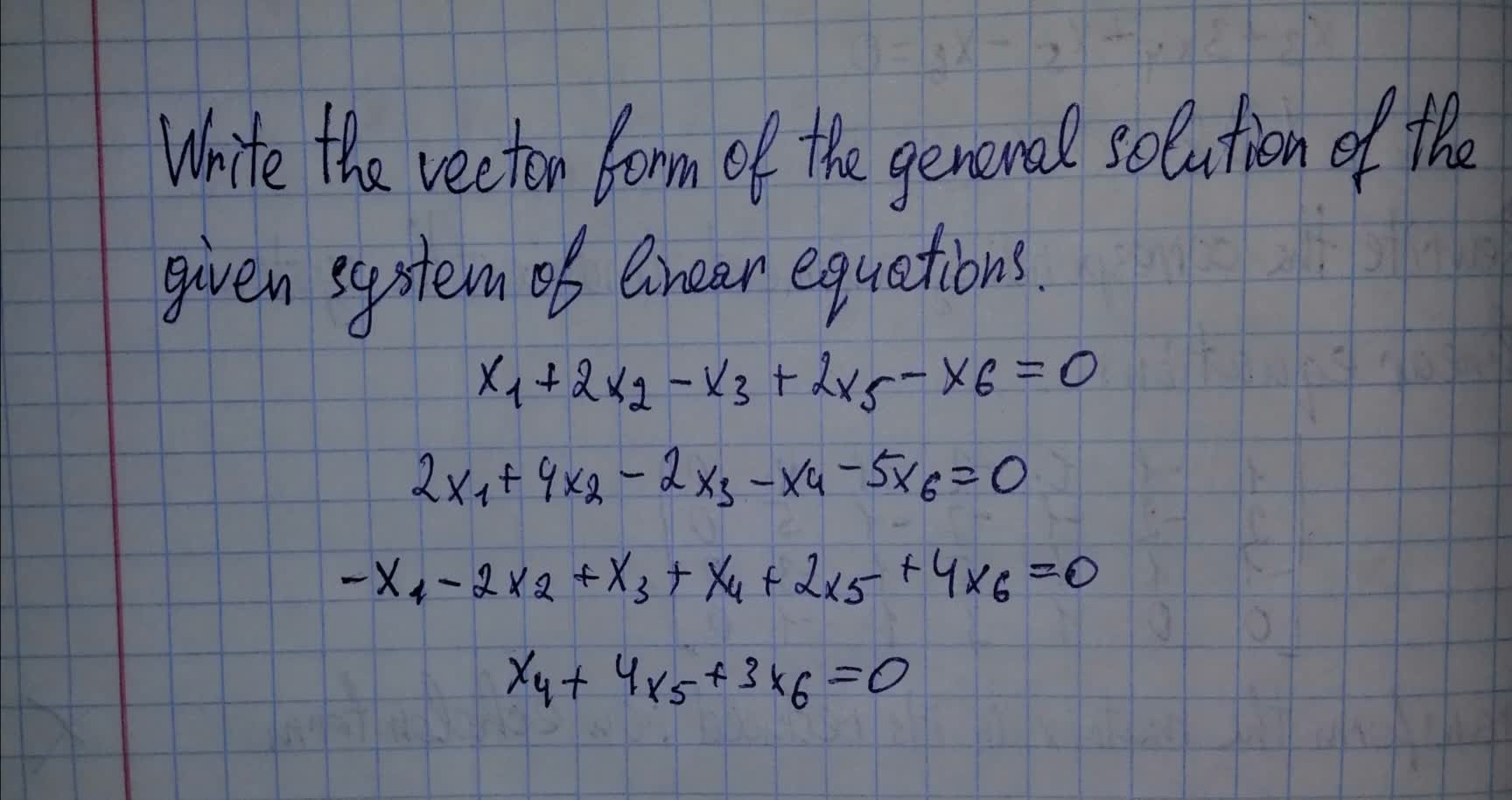Ava-May Nelson 2021-08-11 Answered

### The given matrix is the augmented matrix for a system of linear equations. Give the vector form for the general solution. $$\begin{bmatrix} {1}&{0}&-{1}&-{2}&-{3}&{1}\\{0}&{1}&{2}&{3}&{4}&{0}\end{bmatrix}$$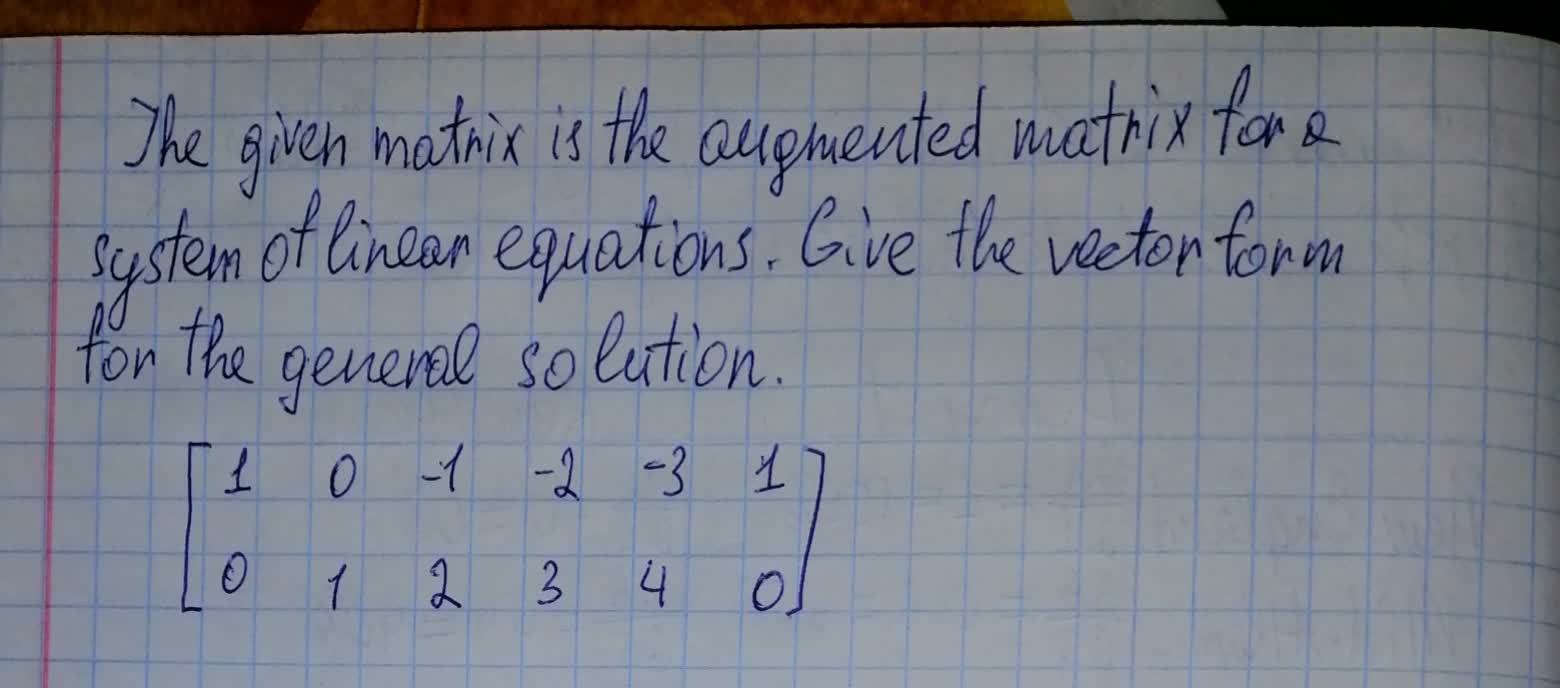Yasmin 2021-08-10 Answered

### The given matrix is the augmented matrix for a system of linear equations. Give the vector form for the general solution. $$\begin{bmatrix}{1}&{0}&-{1}&-{2}&-{3}&{0}\\{0}&{1}&{2}&{3}&{4}&{0}\end{bmatrix}$$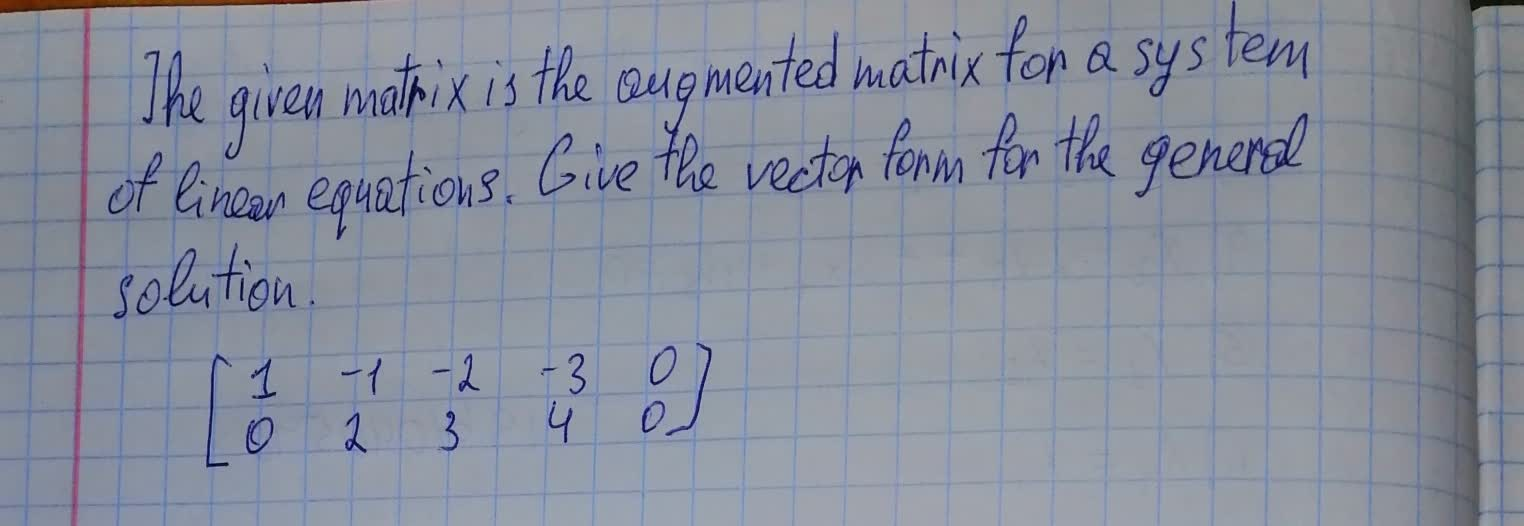sibuzwaW 2021-08-09 Answered

### The reduced row echelon form of the augmented matrix of a system of linear equations is given. Determine whether this system of linear equations is consistent and, if so, find its general solution. $$\begin{bmatrix} {0}&{0}&{1}&-{3}&{0}&{2}&{0}\\ {0}&{0}&{0}&{0}&{1}&-{1}&{0}\\ {0}&{0}&{0}&{0}&{0}&{0}&{0}\\ \end{bmatrix}$$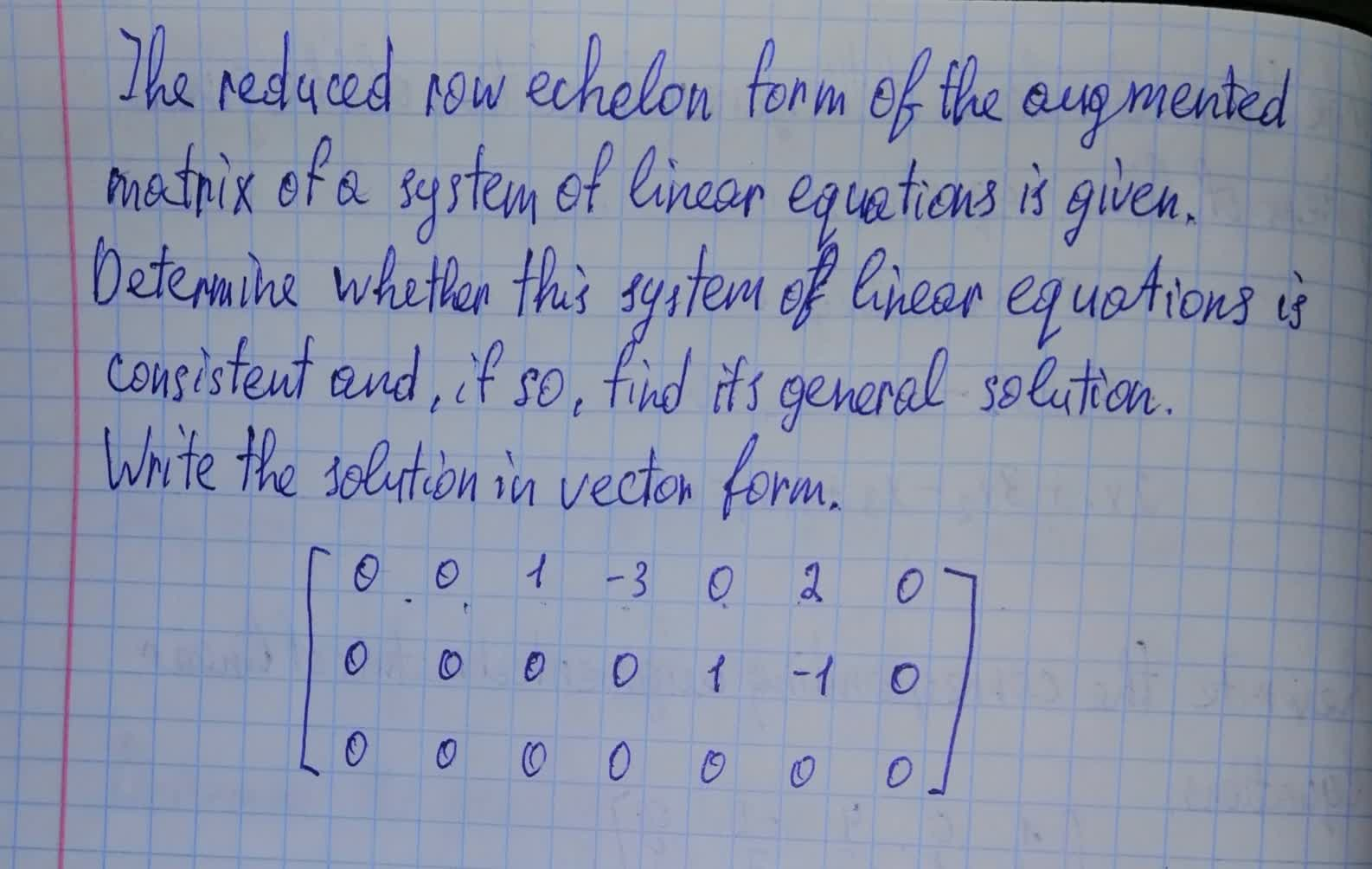illusiia 2021-08-09 Answered

### Write the vector form of the general solution of the given system of linear equations. $$\displaystyle{x}_{{1}}+{4}{x}_{{3}}-{2}{x}_{{4}}={0}$$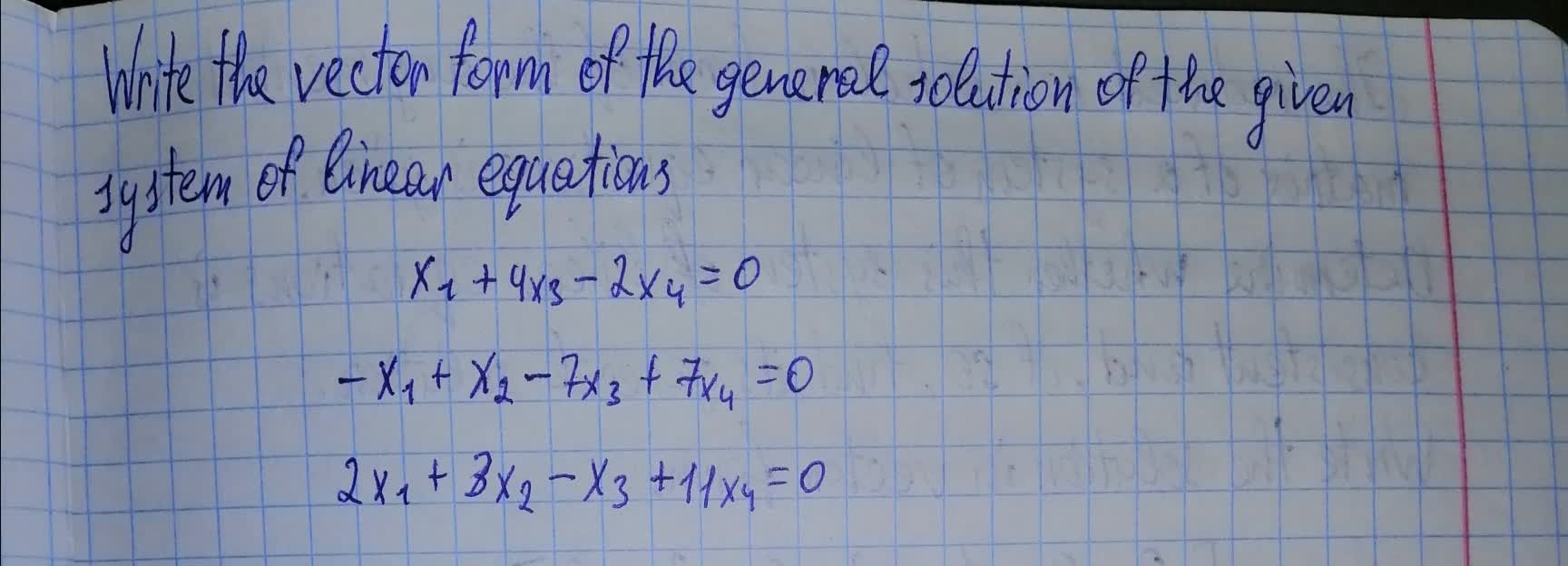texelaare 2021-08-09 Answered

### The coefficient matrix for a system of linear differential equations of the form $$\displaystyle{y}^{{1}}={A}_{{y}}$$ has the given eigenvalues and eigenspace bases. Find the general solution for the system.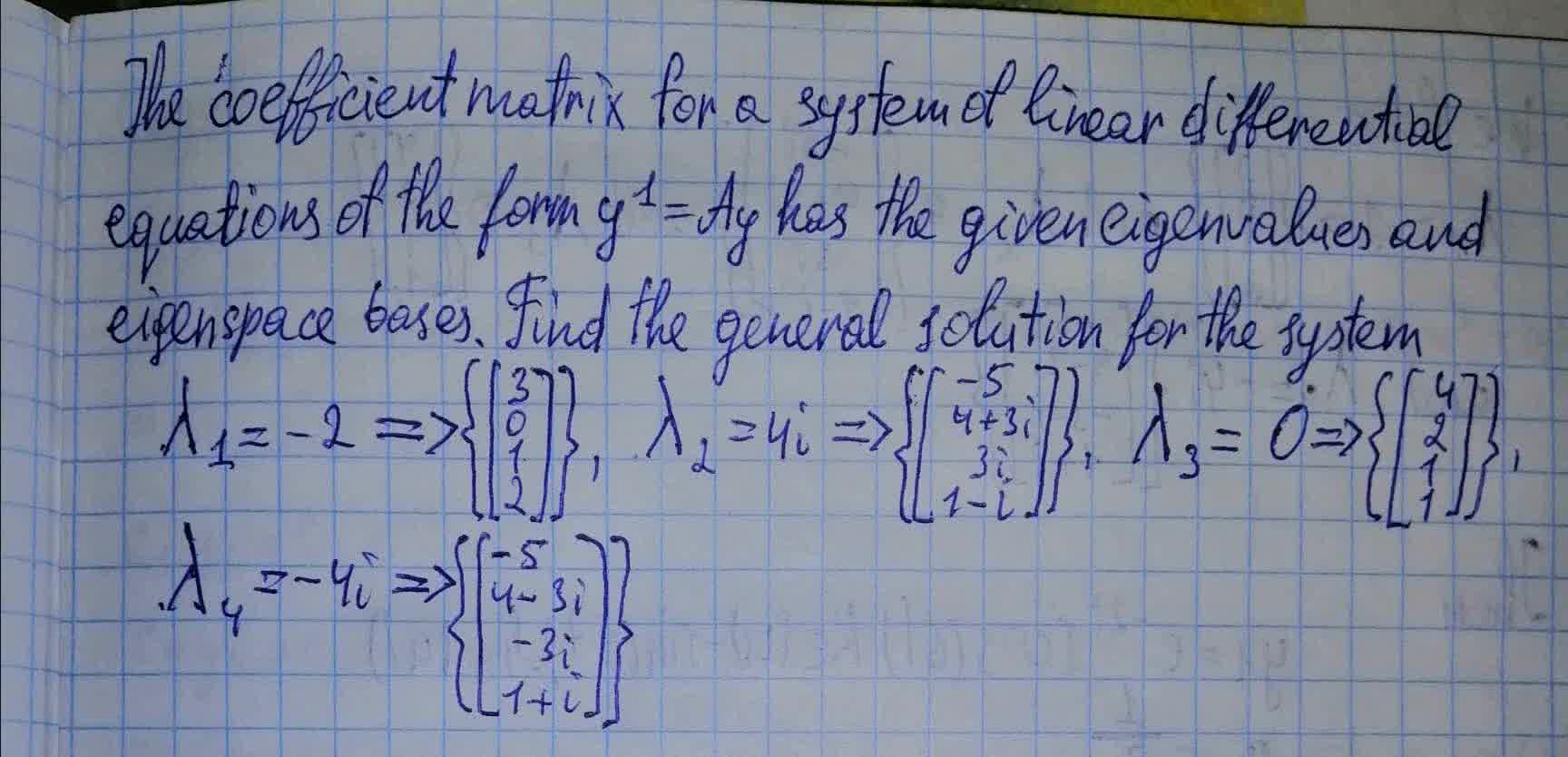lwfrgin 2021-08-08 Answered

### Write the vector form of the general solution of the given system of linear equations. $$\displaystyle{x}_{{1}}-{x}_{{2}}-{2}{x}_{{4}}-{x}_{{5}}+{4}{x}_{{6}}={0}$$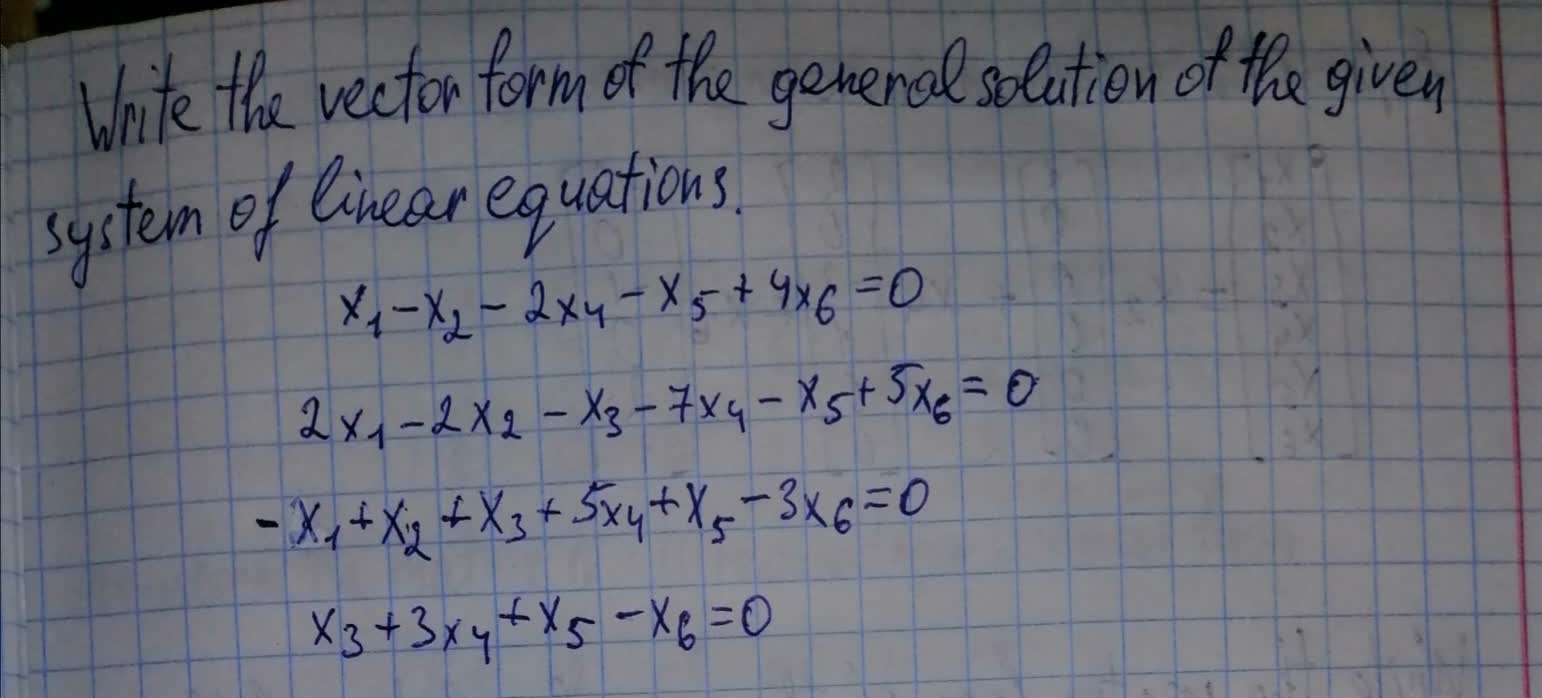Braxton Pugh 2021-08-08 Answered

### The given matrix is the augmented matrix for a system of linear equations $$\Bigg[ \begin{array}{} 1&0&-1&0&-1&-2&0\\ 0&1&2&0&1&2&0 \\ 0&0&0&1&1&1&0\\ \end{array} \Bigg]$$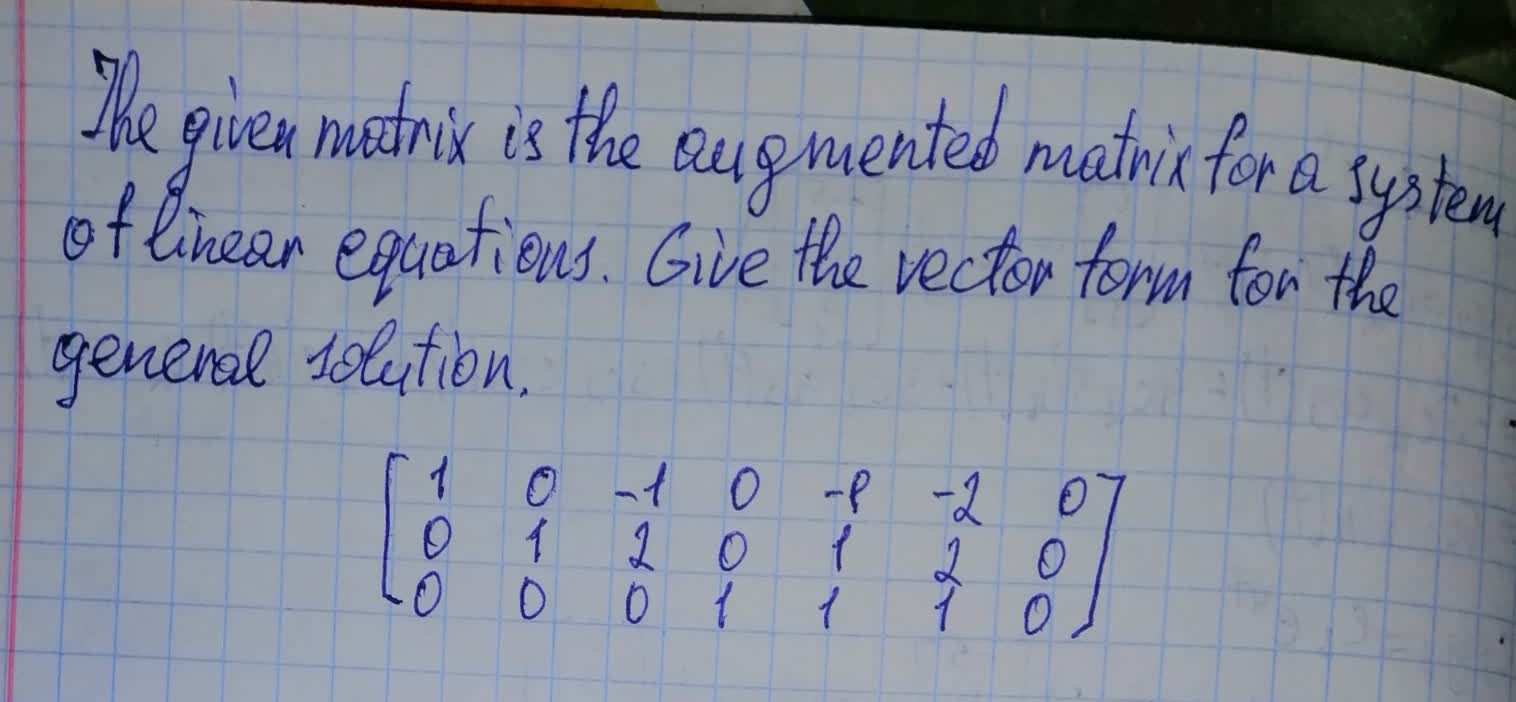boitshupoO 2021-08-07 Answered

### Suppose that the augmented matrix for a system of linear equations has been reduced by row operations to the given row echelon form. Solve the system by back substitution. Assume that the variables are named $$\displaystyle{x}_{{1}},{x}_{{2}},\ldots$$ from left to right. $$\begin{bmatrix}1 & 1&-3&2&1 \\0&1&4&0&3\\0&0&0&1&2 \end{bmatrix}$$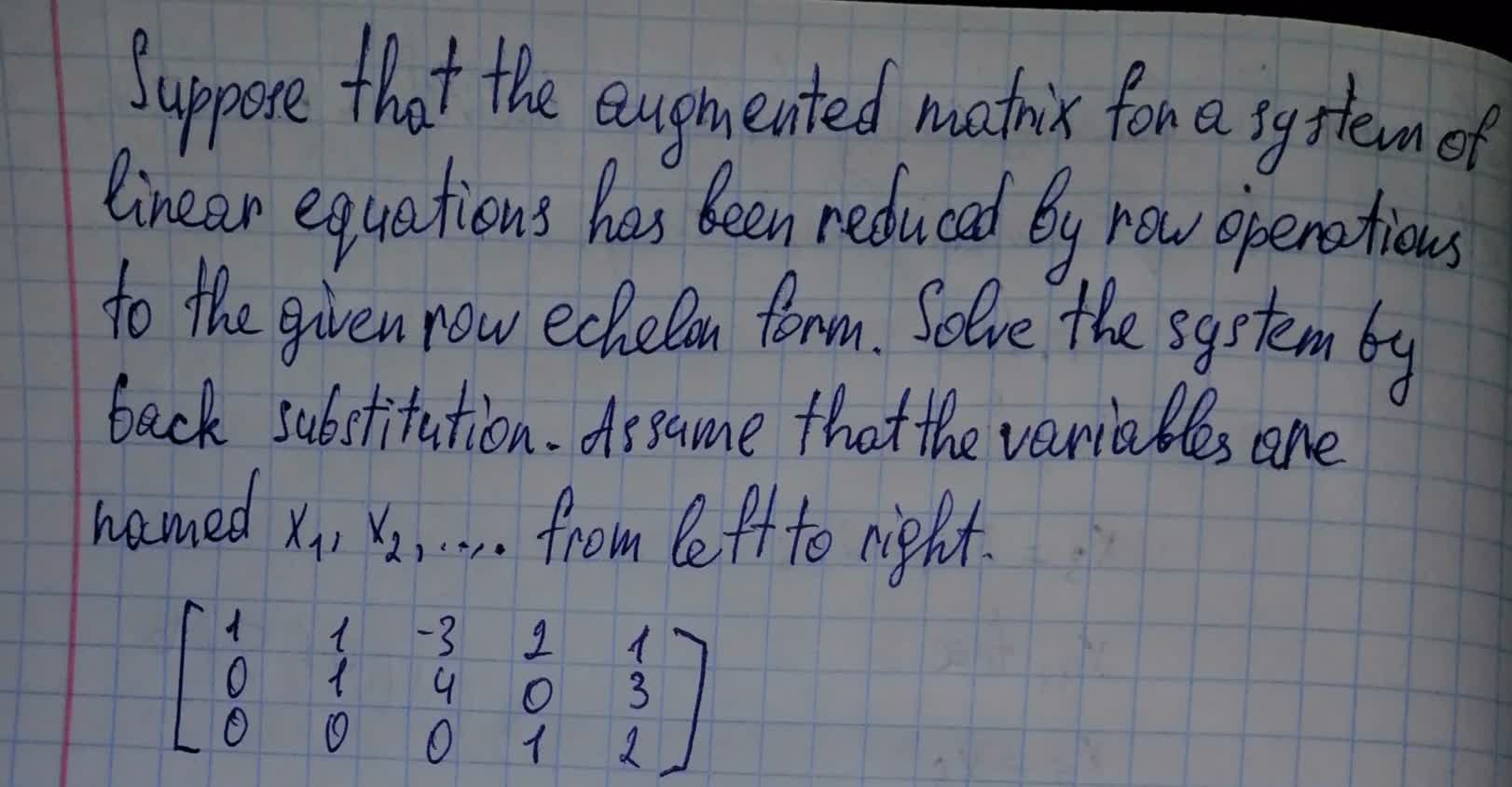Dottie Parra 2021-08-06 Answered

...